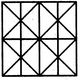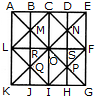# Non Verbal Reasoning - Analytical Reasoning - Discussion

Discussion Forum : Analytical Reasoning - Section 1 (Q.No. 24)
24.

Find the minimum number of straight lines required to make the given figure.11
14
16
17
Explanation:

The figure may be labelled as shown.The horizontal lines are AK, BJ, CI, DH and EG i.e. 5 in number.

The vertical lines are AE, LF and KG i.e. 3 in number.

The slanting lines are LC, CF, FI, LI, EK and AG i.e. 6 in number.

Thus, there are 5 + 3 + 6 = 14 straight lines in the figure.

Discussion:
2 comments Page 1 of 1.

Pallas Athena said:   4 years ago
LC, CF, FI and IL are slanting lines too. You forgot to count them.

Therefore the total number of slanting lines is 6.

K narasimha reddy said:   4 years ago
Correct Answer. Is 10 straight lines.

Here, horizontal lines -3, vertical lines-5, Diagonal lines-2. Total -10.